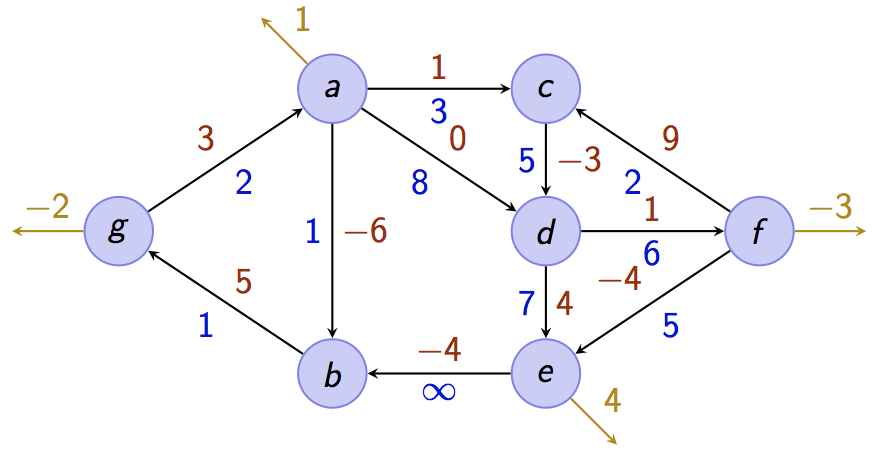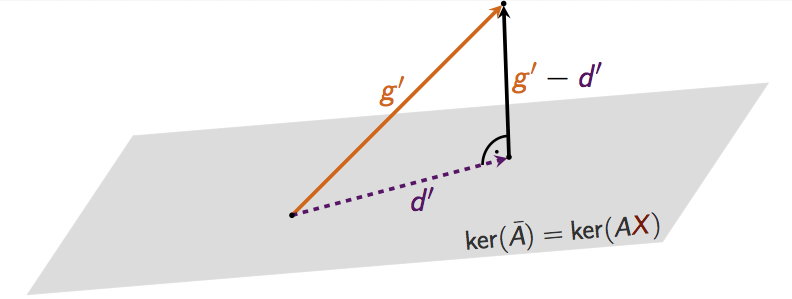max planck institut
informatik# Optimization (Summer Term 2014)

### Basic Information

Lecture time: Tuesday 14:15-16:00 / Thursday 14:15-16:00 HS003 (E1.3) Parinya Chalermsook and Andreas Karrenbauer Ruben Becker; Blaga Davidova, Max John, Andreas Schmid and Daniel Vaz Integer Programming by Laurence A. Wolsey. Introduction to Linear Optimization by Dimitris Bertsimas and John N. Tsitsiklis. Mondays, 2 PM with Andreas in MPII room 024 Tuesdays, 10 AM with Daniel in MPII room 022 Wednesdays, 12 AM with Max in MPII room 023 Fridays, 2 PM with Blaga in MPII room 024

### Announcements

• The Monday tutorial takes place in room 107 in Building E1.3 on May, 5th.
• If you want to participate in the course, please register to our mailinglist.
• The tutorials start on Friday, 25th of April.## Description

This course provides an introduction to fundamental concepts and algorithmic methods for solving linear and integer linear programs.

Linear optimization is a key subject in theoretical computer science. Moreover, it has many applications in practice. A lot of problems can be formulated as (integer) linear optimization problem. For example, combinatorial problems, such as shortest paths, maximum flows, maximum matchings in graphs, among others have a natural formulation as a linear (integer) optimization problem. In this course you will learn:

• how to optimize a linear function subject to linear constraints
• how to formulate combinatorial problems as (integer) linear optimization problems
• how to solve them

To this end, basic concepts from polyhedral theory will be introduced. The simplex algorithm and the ellipsoid method will be presented. The lecture concludes with exact and approximation algorithms for NP-hard optimization problems. There will be theoretical and practical exercises.### Policies

This is a 9-credit-point core lecture ("Stammvorlesung"). There will be two lectures and one exercise session per week. We will hand out exercises every week (usually worth 40 points) and each student should score at least 50% in the first half of the course (first 6 exercise sheets) and 50% in the second half in order to be allowed to take the exam.

You can also gain points by writing lecture notes of single lectures. In order to do so, you have to use our template. You gain $$\gamma\cdot 40$$ points for the notes of one lecture, hereby $$\gamma\in \mathbb{R}$$ denotes the quality factor in between 0 and 1 that will be determined by us. Please submit your notes by sending a zipped package that contains everything such that your tex code compiles and the pdf to Ruben not later than one week after the lecture that you took notes for. You can use the old notes from last year and the source code.

## Lectures

The slides of all lectures are condensed in the handout.

Date Topic Reference Homework Lecturer Notes
Apr 15 Overview, ILP Exercise Sheet 1; Solution 1 Andreas Slides
Apr 17 ILP Chapter 6 in [W] ; Chapter 11.8 in [BT] Andreas Slides; Scribe Notes
Apr 22 Branch and Bound Chapter 7 in [W] ; Chapter 11.2 in [BT] Exercise Sheet 2; Solution 2 Andreas Slides; Scribe Notes
Apr 24 Branch and Bound, Duality Theory see above Andreas Slides; Scribe Notes
Apr 29 Duality Theory Chapter 4.7 in [BT] Exercise Sheet 3; Solution 3 Andreas Slides; Scribe Notes
May 1 No lecture (Labour Day)
May 6 Strong Duality Chapter 4 in [BT] Exercise Sheet 4; Solution 4 Andreas Slides; Scribe Notes
May 8 Polyhedral Theory Chapter 2 in [BT] Andreas Slides; Scribe Notes
May 13 Polyhedral Theory Chapter 2 in [BT] Exercise Sheet 5; Solution 5 Andreas Slides; Scribe Notes
May 15 Simplex Method Chapter 5 in [MG] Parinya Lecture Notes; Scribe Notes
May 20 Simplex Method Chapter 5 in [MG]; Paper by Avis and Kaluzny Exercise Sheet 6; Solution 6 Parinya see lecture notes above; Scribe Notes
May 22 Ellipsoid Method Chapter 3 in [LGS]; Chapter 7 in [MG] Parinya Lecture notes
May 27 Ellipsoid Method Chapter 3 in [LGS]; Chapter 7 in [MG] Exercise Sheet 7; Solution 7 Parinya see lecture notes above
May 29 No lecture (Ascension Day)
June 3 Interior Point Method Chapter 9 in [BT] Exercise Sheet 8; Solution 8 Andreas Slides; Scribe Notes
June 5 Interior Point Method Chapter 9 in [BT] Andreas Slides
June 10 TU Matrices Chapter 3.2 in [W] Exercise Sheet 9; Solution 9 Andreas Slides ; Scribe Notes
June 12 Network Flows Chapter 7.3, 7.4 in [BT]; Chapter 3.3 in [W] Andreas Slides; Scribe Notes
June 17 Network Flows Chapter 7.3, 7.4 in [BT]; Chapter 3.3 in [W] Exercise Sheet 10 Solution 10 Andreas Slides; Scribe Notes
June 19 No lecture (Corpus Christi)
June 24 Matching and Vertex Cover Chapter 8.2 in [MG] Exercise Sheet 11; Solution 11 Parinya Slides ; Scribe Notes
June 26 Minimum Cuts and Shortest Paths Parinya Slides ; Note
July 1 Approximation Algorithms Exercise Sheet 12; Solution 12 Parinya Slides; Scribe Notes
July 3 -- canceled (Makeup class TBD) -- Parinya
July 8 LP/SDP for CSPs Chapter 16.1-16.3 in [VV] Exercise Sheet 13; Solution 13 Parinya Scribe Notes
July 10 LP/SDP for CSPs Chapter 6.1 and 6.2 in [WS] Parinya
July 15 Hierarchies of Convex Relaxation Hierarchies, Knapsack Sample Exam Parinya
July 17 Hierarchies of Convex Relaxation Decomposition Theorem, CT Parinya
July 22 Exam Andreas, Parinya
July 24 Exam Discussion Andreas, Parinya
Prerequisites: Basics in linear algebra, discrete mathematics, calculus, algorithms, and complexity. At Saarland University these topics are covered in the bachelor courses Mathematik für Informatiker 1 & 2, Grundzüge der Theoretischen Informatik, and Grundzüge von Algorithmen und Datenstrukturen.
Exam Information: Your final grade will be the best of the final exam and the re-exam. You may bring one A4 cheat sheet (double-sided, in your own handwriting) to the exams.
Final Exam: July, 22nd 12:00 noon October, 17th at 1:00 pm, in Günter-Hotz-Hörsaal, building E2.2

More Courses of the Algorithms and Complexity Group